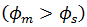# Metal-Semiconductor Junction - Engineering Physics

Metal-Semiconductor Junction

Metal-Semiconductor-Junction is also called hetero-junction because the material on each side of the junction is not the same. These junctions can also produce diodes.

There are two possible types of metal-semiconductor junctions:

- These are the junctions in which work-function of metal is greater than the work-function of a semiconductor.

– These are the junctions in which work-function of metal is less than the work-function of a semiconductor.

Now let us discuss these two types of junctions in detail-

1. Schottky Junction –

One of the first practical semiconductor devices used in the early 1900s was metal-semiconductor diodes. This diode also called a point contact whisper to an exposed semiconductor surface. These metal-semiconductor diodes were not easily reproduced or mechanically reliable and were replaced by the PN junction in the 1950s.

However, semiconductor and vacuum technology is now used to fabricate reproducible and reliable metal-semiconductor contacts. In most cases, the rectifying contacts are made on n-type semiconductor for this reason we will concentrate on this type of diode.

Qualitative Characteristics – The ideal energy-band diagram for a particular metal and n-type semiconductor before making contact is shown in Fig.1. The vacuum level is used as a reference level.

is the metal work-function (measure in volts),is known as electron affinity.

In Figure1, we have assumed thatThe ideal thermal-equilibrium metal-semiconductor energy band diagram, for this situation, is shown in Fig.2.

Before contact, the Fermi level in the semiconductor was above that in the metal. In order for the Fermi level to become a constant through the system in thermal equilibrium, electrons from the semiconductor flow into the lower energy states in the metal. Positively charged donor atoms remain in the semiconductor, creating a space charge region.

is the ideal barrier height of the semiconductor contact, the potential barrier is seen by electrons in the metal trying to move into the semiconductor. This barrier is known as the Schottky barrier and is given by

is the built-in potential barrier. This barrier is the barrier seen by electrons in the conduction band trying to move into the metal. The built-in potential barrier is given by

If a positive voltage is applied to the metal with respect to the semiconductor, the semiconductor-to-metal barrieris reduced whileremains constant. In this situation, electrons can move easily flow from the semiconductor into the metal since the barrier has been reduced. This bias condition is the forward bias. If we apply a positive voltage to the semiconductor with respect to the metal, the semiconductor-to-metal barrier height increases, whileremains constant in this idealized case. This bias condition is the reverse bias. The energy-band diagrams for the forward and reverse bias are shown in Fig.3 and Fig.4, whereis the magnitude of the forward-bias voltage andis the magnitude of the reverse-bias voltage

The Energy-band diagram versus voltage for the metal-semiconductor Junction shown in Fig.3 is very similar to these of the p-n junction. Because of similarity, we expect the current-voltage characteristics of the Schottky barrier junction to be similar to the exponential behavior of the p-n junction diode. In forward bias, the barrier seen by the electrons in the semiconductor is reduced, so the majority charge carrier electrons flow more easily from the semiconductor into the metal. The forward-bias current is in the direction from metal to semiconductor, it is an exponential function of the forward-bias voltageSo act as a rectifying contact.

2. Metal-semiconductor ohmic Junction –

Contacts must be made between any semiconductor device and the outside world. These contacts are made via ohmic contacts. Ohmic contacts are metal-to-semiconductor contacts, but in this case, they are not rectifying contacts. An ohmic contact is low-resistance junction providing conduction in both directions between the metal and the semiconductor. Two general types of ohmic contacts are possible: The first type is the ideal non-rectifying barrier, and the second is the tunneling barrier.

Ideal Non-rectifying Barrier –

In Fig.5 we see the energy levels before contact and in Fig.6, the barrier after contact for thermal equilibrium.

To achieve thermal equilibrium in this junction, electrons will flow from the metal into the lower energy states in the semiconductor, which makes the surface of the semiconductor more n-type. The excess electron charge in the n-type semiconductor exists essentially as a surface charge density. If a positive voltage is applied to the metal, there is no barrier to electrons flowing from the semiconductor into the metal. If a positive voltage is applied to the semiconductor, the effective barrier height for electrons flowing from the metal into the semiconductor will be approximatelywhich is fairly small for a moderately to the heavily doped semiconductor. For this bias condition, electrons can easily flow from the metal into the semiconductor. Fig.7 shows the energy band diagram. When a positive voltage is applied to the metal with respect to the semiconductor. Electrons can easily flow “down-hill” from the semiconductor into the metal. Fig.8 shows the case when a positive voltage is applied to the semiconductor with respect to the metal. The electron can easily flow over the barrier from the metal into the semiconductor. This junction, thus, is an ohmic contact.

Tunneling Barrier –

The space charge within a rectifying metal-semiconductor contact is inversely proportional to the square root of the semiconductor doping. The width of the depletion region decreases as the doping concentration increases. The probability to tunneling through the barrier.

Fig.9 shows a junction in which the metal is in contact with a heavily doped n-type epitaxial layer.

The tunneling current has the form

Where

The tunneling current increases exponentially with doping concentration.

1.2.